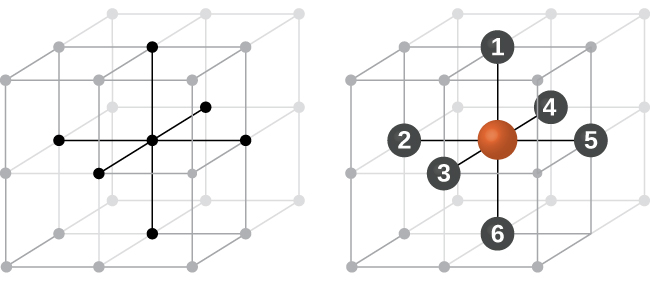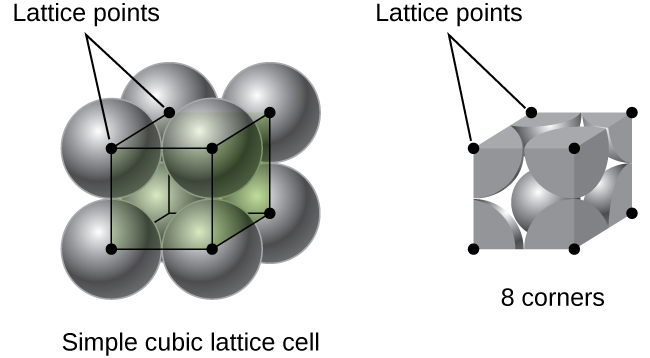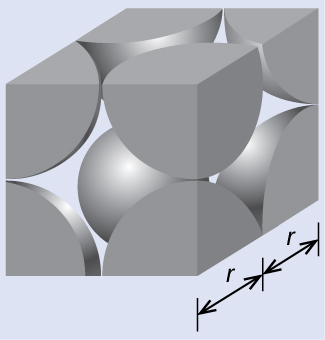# 10.6 Lattice structures in crystalline solids  (Page 2/29)

 Page 2 / 29An atom in a simple cubic lattice structure contacts six other atoms, so it has a coordination number of six.

In a simple cubic lattice, the unit cell that repeats in all directions is a cube defined by the centers of eight atoms, as shown in [link] . Atoms at adjacent corners of this unit cell contact each other, so the edge length of this cell is equal to two atomic radii, or one atomic diameter. A cubic unit cell contains only the parts of these atoms that are within it. Since an atom at a corner of a simple cubic unit cell is contained by a total of eight unit cells, only one-eighth of that atom is within a specific unit cell. And since each simple cubic unit cell has one atom at each of its eight “corners,” there is $8\phantom{\rule{0.2em}{0ex}}×\phantom{\rule{0.2em}{0ex}}\frac{1}{8}\phantom{\rule{0.2em}{0ex}}=1$ atom within one simple cubic unit cell.A simple cubic lattice unit cell contains one-eighth of an atom at each of its eight corners, so it contains one atom total.

## Calculation of atomic radius and density for metals, part 1

The edge length of the unit cell of alpha polonium is 336 pm.

(a) Determine the radius of a polonium atom.

(b) Determine the density of alpha polonium.

## Solution

Alpha polonium crystallizes in a simple cubic unit cell:(a) Two adjacent Po atoms contact each other, so the edge length of this cell is equal to two Po atomic radii: l = 2 r . Therefore, the radius of Po is $r\phantom{\rule{0.2em}{0ex}}=\phantom{\rule{0.2em}{0ex}}\frac{\text{l}}{2}\phantom{\rule{0.2em}{0ex}}=\phantom{\rule{0.2em}{0ex}}\frac{\text{336 pm}}{2}\phantom{\rule{0.2em}{0ex}}=\text{168 pm}.$

(b) Density is given by $\text{density}\phantom{\rule{0.2em}{0ex}}=\phantom{\rule{0.2em}{0ex}}\frac{\text{mass}}{\text{volume}}.$ The density of polonium can be found by determining the density of its unit cell (the mass contained within a unit cell divided by the volume of the unit cell). Since a Po unit cell contains one-eighth of a Po atom at each of its eight corners, a unit cell contains one Po atom.

The mass of a Po unit cell can be found by:

$\text{1 Po unit cell}\phantom{\rule{0.2em}{0ex}}×\phantom{\rule{0.2em}{0ex}}\frac{\text{1 Po atom}}{\text{1 Po unit cell}}\phantom{\rule{0.2em}{0ex}}×\phantom{\rule{0.2em}{0ex}}\frac{\text{1 mol Po}}{6.022\phantom{\rule{0.2em}{0ex}}×\phantom{\rule{0.2em}{0ex}}{10}^{23}\phantom{\rule{0.2em}{0ex}}\text{Po atoms}}\phantom{\rule{0.2em}{0ex}}×\phantom{\rule{0.2em}{0ex}}\frac{208.998\phantom{\rule{0.2em}{0ex}}\text{g}}{\text{1 mol Po}}\phantom{\rule{0.2em}{0ex}}=3.47\phantom{\rule{0.2em}{0ex}}×\phantom{\rule{0.2em}{0ex}}{10}^{-22}\phantom{\rule{0.2em}{0ex}}\text{g}$

The volume of a Po unit cell can be found by:

$V={l}^{3}={\left(336\phantom{\rule{0.2em}{0ex}}×\phantom{\rule{0.2em}{0ex}}{10}^{-10}\phantom{\rule{0.2em}{0ex}}\text{cm}\right)}^{3}=3.79\phantom{\rule{0.2em}{0ex}}×\phantom{\rule{0.2em}{0ex}}{10}^{-23}\phantom{\rule{0.2em}{0ex}}{\text{cm}}^{3}$

(Note that the edge length was converted from pm to cm to get the usual volume units for density.)

Therefore, the density of $\text{Po}=\phantom{\rule{0.2em}{0ex}}\frac{3.471\phantom{\rule{0.2em}{0ex}}×\phantom{\rule{0.2em}{0ex}}{10}^{-22}\phantom{\rule{0.2em}{0ex}}\text{g}}{3.79\phantom{\rule{0.2em}{0ex}}×\phantom{\rule{0.2em}{0ex}}{10}^{-23}\phantom{\rule{0.2em}{0ex}}{\text{cm}}^{3}}\phantom{\rule{0.2em}{0ex}}={\text{9.16 g/cm}}^{3}$

The edge length of the unit cell for nickel is 0.3524 nm. The density of Ni is 8.90 g/cm 3 . Does nickel crystallize in a simple cubic structure? Explain.

No. If Ni was simple cubic, its density would be given by:
$\text{1 Ni atom}\phantom{\rule{0.2em}{0ex}}×\phantom{\rule{0.2em}{0ex}}\frac{\text{1 mol Ni}}{6.022\phantom{\rule{0.2em}{0ex}}×\phantom{\rule{0.2em}{0ex}}{10}^{23}\phantom{\rule{0.2em}{0ex}}\text{Ni atoms}}\phantom{\rule{0.2em}{0ex}}×\phantom{\rule{0.2em}{0ex}}\frac{58.693\phantom{\rule{0.2em}{0ex}}\text{g}}{\text{1 mol Ni}}\phantom{\rule{0.2em}{0ex}}=9.746\phantom{\rule{0.2em}{0ex}}×\phantom{\rule{0.2em}{0ex}}{10}^{-23}\phantom{\rule{0.2em}{0ex}}\text{g}$
$V={l}^{3}={\left(3.524\phantom{\rule{0.2em}{0ex}}×\phantom{\rule{0.2em}{0ex}}{10}^{-8}\phantom{\rule{0.2em}{0ex}}\text{cm}\right)}^{3}=4.376\phantom{\rule{0.2em}{0ex}}×\phantom{\rule{0.2em}{0ex}}{10}^{-23}\phantom{\rule{0.2em}{0ex}}{\text{cm}}^{3}$
Then the density of Ni would be $=\phantom{\rule{0.2em}{0ex}}\frac{9.746\phantom{\rule{0.2em}{0ex}}×\phantom{\rule{0.2em}{0ex}}{10}^{-23}\phantom{\rule{0.2em}{0ex}}\text{g}}{4.376\phantom{\rule{0.2em}{0ex}}×\phantom{\rule{0.2em}{0ex}}{10}^{-23}\phantom{\rule{0.2em}{0ex}}{\text{cm}}^{3}}\phantom{\rule{0.2em}{0ex}}={\text{2.23 g/cm}}^{3}$
Since the actual density of Ni is not close to this, Ni does not form a simple cubic structure.

Most metal crystals are one of the four major types of unit cells. For now, we will focus on the three cubic unit cells: simple cubic (which we have already seen), body-centered cubic unit cell    , and face-centered cubic unit cell    —all of which are illustrated in [link] . (Note that there are actually seven different lattice systems, some of which have more than one type of lattice, for a total of 14 different types of unit cells. We leave the more complicated geometries for later in this module.)

what is the meaninh of link
Yes wish to join am a science ⚗ student
What is acid
Emmanuel
is agroup of substance that relaise hydrogen ion in the water solution.
mengistu
thanks
Aisha
An acid is a proton donor or an electron pair acceptor
amanda
how can someone understand chemistry vividly
Maybe by reading proofs or practical work and application in modern world.
Abdelkarim
what is isotopes
whats de shape of water
water has no shape because it's liquid
Wil
water is a shapeless, odourless, colourless and tasteless substance that only takes the shape of its container.
mikefred
i think they're referring to the molecular shape?
It has no shape but takes the shape of the container
Yeah I think so too and it's a bent shape
amanda
what isthe maening pkw
what is the meaning of STP
Laura
introduction to chemistry for beginner
hi
noble
through out human history
Yes?
Esther
hello
noble
yes
Bol
what
noble
is euglena a unicellular organ
is euglena a unicellular organism
Agio
how is hydrogen can be heated
what is difference between atom and molecule
Atom is the smallest part of matter; it consists of equal number of protons and electrons. It may have neutrons. A molecule is a compound made of atoms covalently bonded.
Abdelkarim
does amoeba has structure
what is the effect of green house on the earth 🌎
what is the effect of green house on the earth 🌎
kolawole
what's an atom?
it's the smallest unit of Matter
Orsine
atom
Eden
smallest part of an element
lydia
also, depending on its (atom's) structure, that is the amount of protons and neutrons and electrons, is the determining factors of what element it is.
Richard
is a smallast particals of an element
Buwembo
it is the smallest part of an element that can take part in a chemical reaction
Ayub
is the smallest part of an element
Jonathan
An atom is the smallest indivisible part of a matter
Oy kl konsa test hay or kitna hay?

#### Get Jobilize Job Search Mobile App in your pocket Now!ByByBy Madison ChristianBy Jessica CollettBy Dindin SecretoBy RhodesBy JavaChamp TeamBy Tamsin KnoxBy OpenStaxBy Jordon HumphreysBy Richley CrapoBy OpenStax